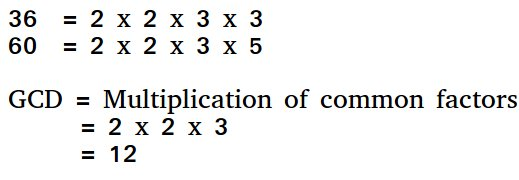Program to find HCF (Highest Common Factor) of 2 Numbers

• Last Updated : 06 Oct, 2021

HCF (Highest Common Factor) or GCD (Greatest Common Divisor) of two numbers is the largest number that divides both of them.For example GCD of 20 and 28 is 4 and GCD of 98 and 56 is 14.

A simple solution is to find all prime factors of both numbers, then find intersection of all factors present in both numbers. Finally return product of elements in the intersection.

An efficient solution is to use Euclidean algorithm which is the main algorithm used for this purpose. The idea is, GCD of two numbers doesn’t change if smaller number is subtracted from a bigger number.

C++

 // C++ program to find GCD of two numbers#include using namespace std; // Recursive function to return gcd of a and bint gcd(int a, int b){       // Everything divides 0    if (a == 0 && b == 0)        return 0;    if (a == 0)        return b;    if (b == 0)        return a;     // base case    if (a == b)        return a;     // a is greater    if (a > b)        return gcd(a - b, b);    return gcd(a, b - a);} // Driver program to test above functionint main(){    int a = 0, b = 56;    cout << "GCD of " << a << " and " << b <<  " is " << gcd(a, b);    return 0;} // This code is contributed by shivanisinghss2110

C

 // C program to find GCD of two numbers#include  // Recursive function to return gcd of a and bint gcd(int a, int b){    // Everything divides 0    if (a == 0 && b == 0)        return 0;    if (a == 0)        return b;    if (b == 0)        return a;     // base case    if (a == b)        return a;     // a is greater    if (a > b)        return gcd(a - b, b);    return gcd(a, b - a);} // Driver program to test above functionint main(){    int a = 0, b = 56;    printf("GCD of %d and %d is %d ", a, b, gcd(a, b));    return 0;}

Java

 // Java program to find GCD of two numbersclass Test {    // Recursive function to return gcd of a and b    static int gcd(int a, int b)    {        // Everything divides 0        if (a == 0 && b == 0)            return 0;        if (a == 0)            return b;        if (b == 0)            return a;         // base case        if (a == b)            return a;         // a is greater        if (a > b)            return gcd(a - b, b);        return gcd(a, b - a);    }     // Driver method    public static void main(String[] args)    {        int a = 98, b = 56;        System.out.println("GCD of " + a + " and " + b                           + " is " + gcd(a, b));    }}

Python3

 # Recursive function to return gcd of a and bdef gcd(a, b):     # Everything divides 0    if(a == 0 and b == 0):        return 0           if(a == 0):        return b         if(b == 0):        return a         # base case    if(a == b):        return a     # a is greater    if (a > b):        return gcd(a-b, b)    return gcd(a, b-a) # Driver program to test above functiona = 98b = 56if(gcd(a, b)):    print('GCD of', a, 'and', b, 'is', gcd(a, b))else:    print('not found') # This code is contributed by Danish Raza

C#

 // C# program to find GCD of two// numbersusing System; class GFG {     // Recursive function to return    // gcd of a and b    static int gcd(int a, int b)    {         // Everything divides 0        if (a == 0 && b == 0)            return 0;        if (a == 0)            return b;        if (b == 0)            return a;         // base case        if (a == b)            return a;         // a is greater        if (a > b)            return gcd(a - b, b);         return gcd(a, b - a);    }     // Driver method    public static void Main()    {        int a = 98, b = 56;        Console.WriteLine("GCD of " + a + " and " + b                          + " is " + gcd(a, b));    }} // This code is contributed by anuj_67.

PHP

 \$b)        return gcd( \$a-\$b , \$b ) ;     return gcd( \$a , \$b-\$a ) ;} // Driver code\$a = 98 ;\$b = 56 ; echo "GCD of \$a and \$b is ", gcd(\$a , \$b) ; // This code is contributed by Anivesh Tiwari?>

Javascript



Output:

GCD of 98 and 56 is 14

A more efficient solution is to use modulo operator in Euclidean algorithm

C++

 // C++ program to find GCD of two numbers#include using namespace std; // Recursive function to return gcd of a and bint gcd(int a, int b){    if (b == 0)        return a;    return gcd(b, a % b);} // Driver program to test above functionint main(){    int a = 98, b = 56;    cout<<"GCD of " <

C

 // C program to find GCD of two numbers#include  // Recursive function to return gcd of a and bint gcd(int a, int b){    if (b == 0)        return a;    return gcd(b, a % b);} // Driver program to test above functionint main(){    int a = 98, b = 56;    printf("GCD of %d and %d is %d ", a, b, gcd(a, b));    return 0;}

Java

 // Java program to find GCD of two numbersclass Test{    // Recursive function to return gcd of a and b    static int gcd(int a, int b)    {      if (b == 0)        return a;      return gcd(b, a % b);    }         // Driver method    public static void main(String[] args)    {        int a = 98, b = 56;        System.out.println("GCD of " + a +" and " + b +                                   " is " + gcd(a, b));    }}

Python3

 # Recursive function to return gcd of a and bdef gcd(a,b):         # Everything divides 0    if (b == 0):         return a    return gcd(b, a%b) # Driver program to test above functiona = 98b = 56if(gcd(a, b)):    print('GCD of', a, 'and', b, 'is', gcd(a, b))else:    print('not found') # This code is contributed by Danish Raza

C#

 // C# program to find GCD of two// numbersusing System; class GFG {         // Recursive function to return    // gcd of a and b    static int gcd(int a, int b)    {            if (b == 0)          return a;       return gcd(b, a % b);    }         // Driver method    public static void Main()    {        int a = 98, b = 56;        Console.WriteLine("GCD of "          + a +" and " + b + " is "                      + gcd(a, b));    }} // This code is contributed by anuj_67.



Javascript



Output:

GCD of 98 and 56 is 14

Please refer GCD of more than two (or array) numbers to find HCF of more than two numbers.